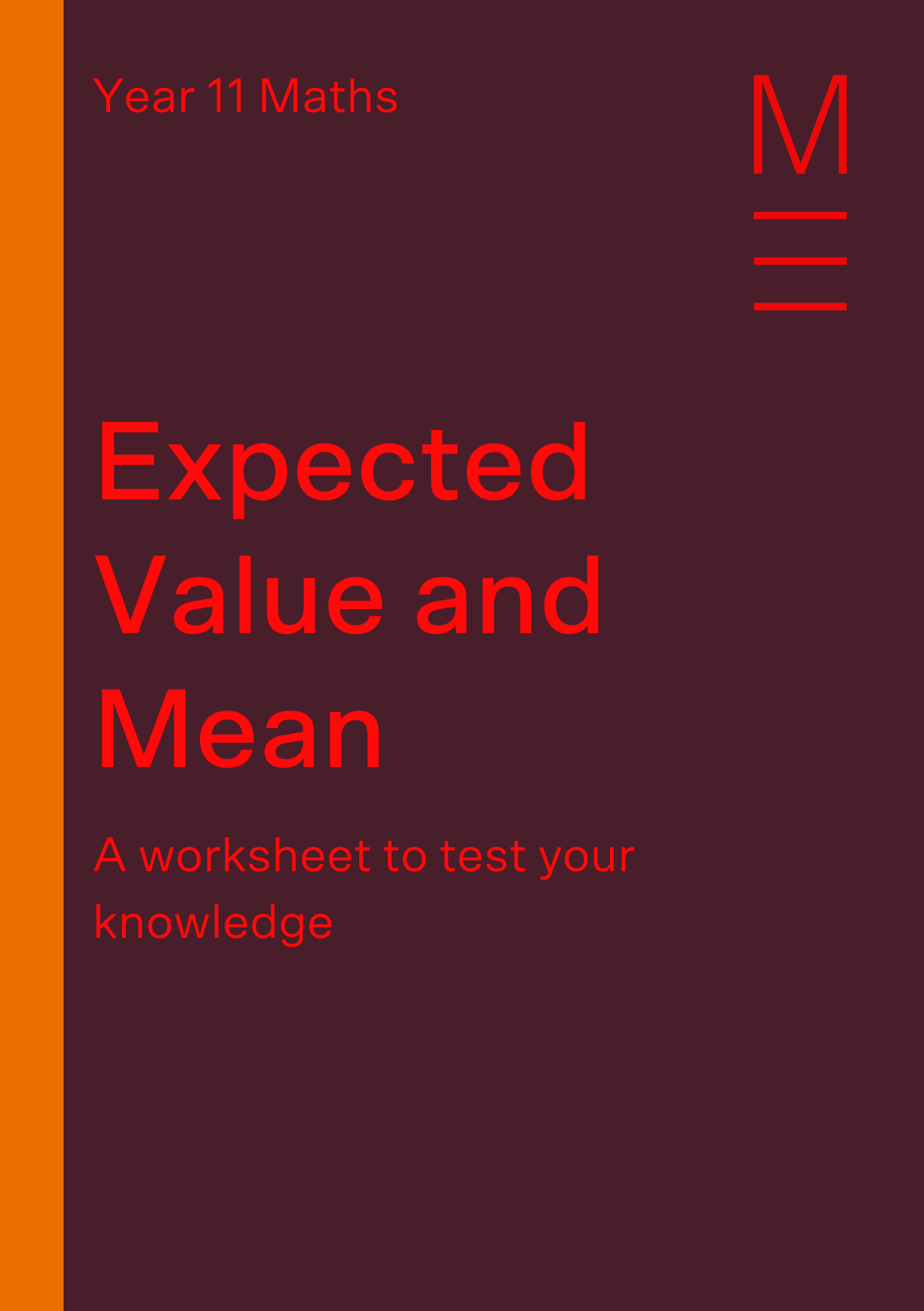# Part 10: Expected Value and Mean | Year 11 Statistics

Finally, got the hang of discrete random variables? Well, let's use that knowledge to ace expected values and mean!Today we’ll be delving deeper into discrete random variables and investigating the expected value and mean, working out how to calculate them and interpreting the results.

## Year 11 Advanced Mathematics: Expected Value and Mean

We want to find characteristics about the data and probability in order to understand their relationship. One of these is the expected value or mean, which can give us information about a typical scenario. This is incredibly useful information for anyone who may be projecting into the future, giving you a sense of what may lie ahead so you can plan for it.

## NESA Syllabus Outcomes

Let’s have a closer look at what the Stage 6 Mathematics Advanced syllabus asks of us.

### S1.2 Discrete Probability Distributions

• Use discrete random variables and associated probabilities to solve practical problems
• Recognise the mean or expected value, $$?(?) = ?$$, of a discrete random variable $$?$$ as a measure of centre, and evaluate it in simple casesTest your knowledge of Expected Value and Mean

Develop your knowledge and statistics skills with this free worksheet.

## Assumed Knowledge

You should hopefully be familiar with discrete random variables by now and be comfortable working with a probability distribution. If not, take a couple minutes to review this.
It’s also important to be familiar with sigma notation.

## Expected Value and Mean

The expected value is one measure of centrality. It is also known as the mean, a term you may already be familiar with.

We can calculate the expected value using the following formula:

$$\sum_{i=0}^{n}x_i P(x_i)$$

This asks us to add the products of all the possible values our variable can take and their corresponding probabilities.

### Example:

A meteorologist is trying to predict what the temperature might be like in Sydney tomorrow.

His computer tells him that there is a $$20%$$ chance of it being $$12$$ degrees, $$30%$$ chance of $$14$$ degrees and $$50%$$ of $$16$$ degrees.

What is the expected temperature for tomorrow?

 $$X$$ $$12$$ $$14$$ $$16$$ $$P(X=x)$$ $$20$$ $$30$$ $$50$$ $$X \times P(X=x)$$ $$2.4$$ $$4.2$$ $$8.0$$

Multiply each temperature here with its corresponding probability, and sum each result up.
$$E(x)=0.2(12)+0.3(14)+0.5(16)=14.6$$
Therefore, the expected temperature tomorrow is $$14.6 ºC$$, a bit chilly if you ask me.

One of the more creative ways we can extended this principle is in the world of betting and games. Calculating our expected value could potentially guide us whether we could win or lose money playing these games.

If $$E(x)>0$$, we should expect to win money from playing
If $$E(x)<0$$, we should expect to lose money from playing
If $$E(x)=0$$, we should break-even after playing

## Want to make sure your Year 11 Maths Advanced results show the expected value?

Boost your Band 5 marks into Band 6s!

Clear, structured Theory Lesson Videos and one-to-one help to give you exam confidence.## Concept Check Questions

1. Calculate the mean from the following probability distribution

 $$X$$ $$1$$ $$3$$ $$5$$ $$7$$ $$P(X=x)$$ $$0.2$$ $$0.5$$ $$0.15$$ $$0.15$$

2. Sam is playing a game where he bets on the face of a card from a standard $$52$$ card deck. Each entry to play is $$2$$. He wins $$1$$ if a number appears, $$3$$ if a royal appears and $$8$$ for an ace to appear. How much could he expect to win if he plays five times?

3. Helen and Maxine have counted three blue cars, four grey cars and two white cars on their street. Helen will pay Maxine $$1$$ if a blue car leaves first, $$3$$ for a grey car and $$2.50$$ for a white car. What can Maxine expect her payout to be, on average?

 $$X$$ $$1$$ $$3$$ $$2.50$$ $$P(X=x)$$ $$\frac{3}{9}$$ $$\frac{4}{9}$$ $$\frac{3}{9}$$ $$X \times P(X=x)$$ $$\frac{3}{9}$$ $$\frac{12}{9}$$ $$\frac{5}{6}$$

4. Find the values of $$\alpha$$ and $$\beta$$, given that the mean for this probability distribution is $$3.25$$.

 $$X$$ $$2$$ $$3$$ $$4$$ $$5$$ $$P(X=x)$$ $$\alpha$$ $$\beta$$ $$0.2$$ $$0.25$$

5. Calculate the mean from this probability distribution

 $$X$$ $$1$$ $$8$$ $$6$$ $$5$$ $$P(X=x)$$ $$3\alpha$$ $$2\alpha$$ $$0.2$$ $$0.25$$

## Concept Check Solutions

1. $$E(x)=0.2(1)+0.5(3)+0.15(5)+0.15(7)=3.5$$

2.

 $$X$$ $$1$$ $$3$$ $$8$$ $$P(X=x)$$ $$\frac{36}{52}$$ $$\frac{12}{52}$$ $$\frac{4}{52}$$ $$X \times P(X=x)$$ $$\frac{36}{52}$$ $$\frac{36}{52}$$ $$\frac{32}{52}$$
$$E(x)=\frac{36}{52}+\frac{36}{52}+\frac{32}{52}=2$$

Since the entry cost to play is $$2$$, Sam would therefore break even from playing this game.

3. $$E(x)=\frac{3}{9}+\frac{12}{9}+\frac{5}{6}=2.50$$

4. $$\alpha=0.45$$ and $$\beta=0.1$$

5. $$E(x)=1(0.33)+8(0.22)+6(0.2)+5(0.25)=4.54$$

© Matrix Education and www.matrix.edu.au, 2022. Unauthorised use and/or duplication of this material without express and written permission from this site’s author and/or owner is strictly prohibited. Excerpts and links may be used, provided that full and clear credit is given to Matrix Education and www.matrix.edu.au with appropriate and specific direction to the original content.